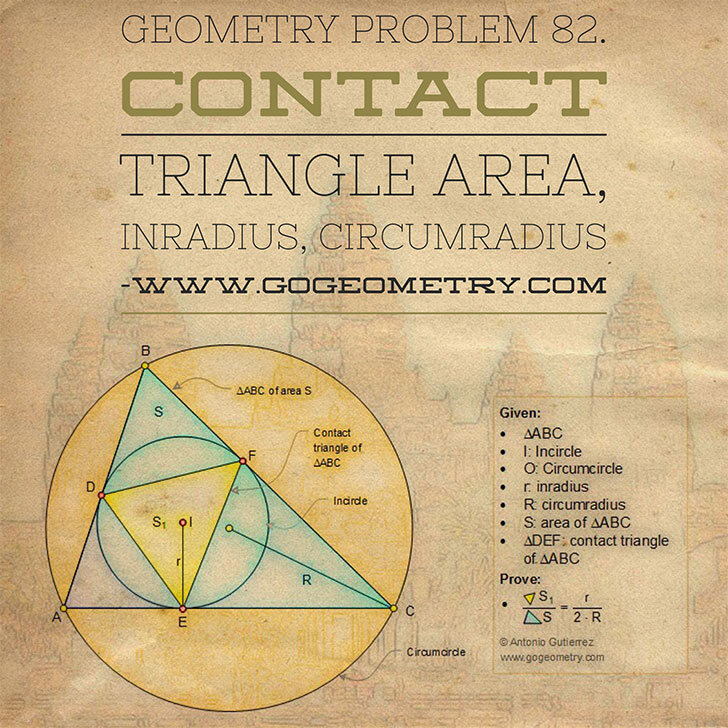# Geometry Problem 82. Area of the Contact Triangle, Inradius, Circumradius. High School, College, Math Education

 Given a triangle ABC of area S, the incircle of center I, the inradius r, and the circumradius R. If S1 is the area of the contact triangle DEF, prove that: $$\dfrac{S_1}{S}=\dfrac{r}{2\cdot R}$$.See also: Original problem 82 art Kaleidoscope problem 82  HINTS: See: Problem 81. CONTACT TRIANGLE: The contact triangle of a triangle ABC (figure above), also called the intouch triangle, is the triangle DEF formed by the points of tangency of the incircle of triangle ABC with triangle ABC.   AREA OF A TRIANGLE: Semiperimeter and Inradius Formula## Poster of Problem 82: Sketching, Typography, Art, iPad ProHome | Search | Geometry | Problems | All Problems | Open Problems | Visual Index | Art | 81-90 | Circle Tangent Line | Email | View or post a solution | by Antonio Gutierrez